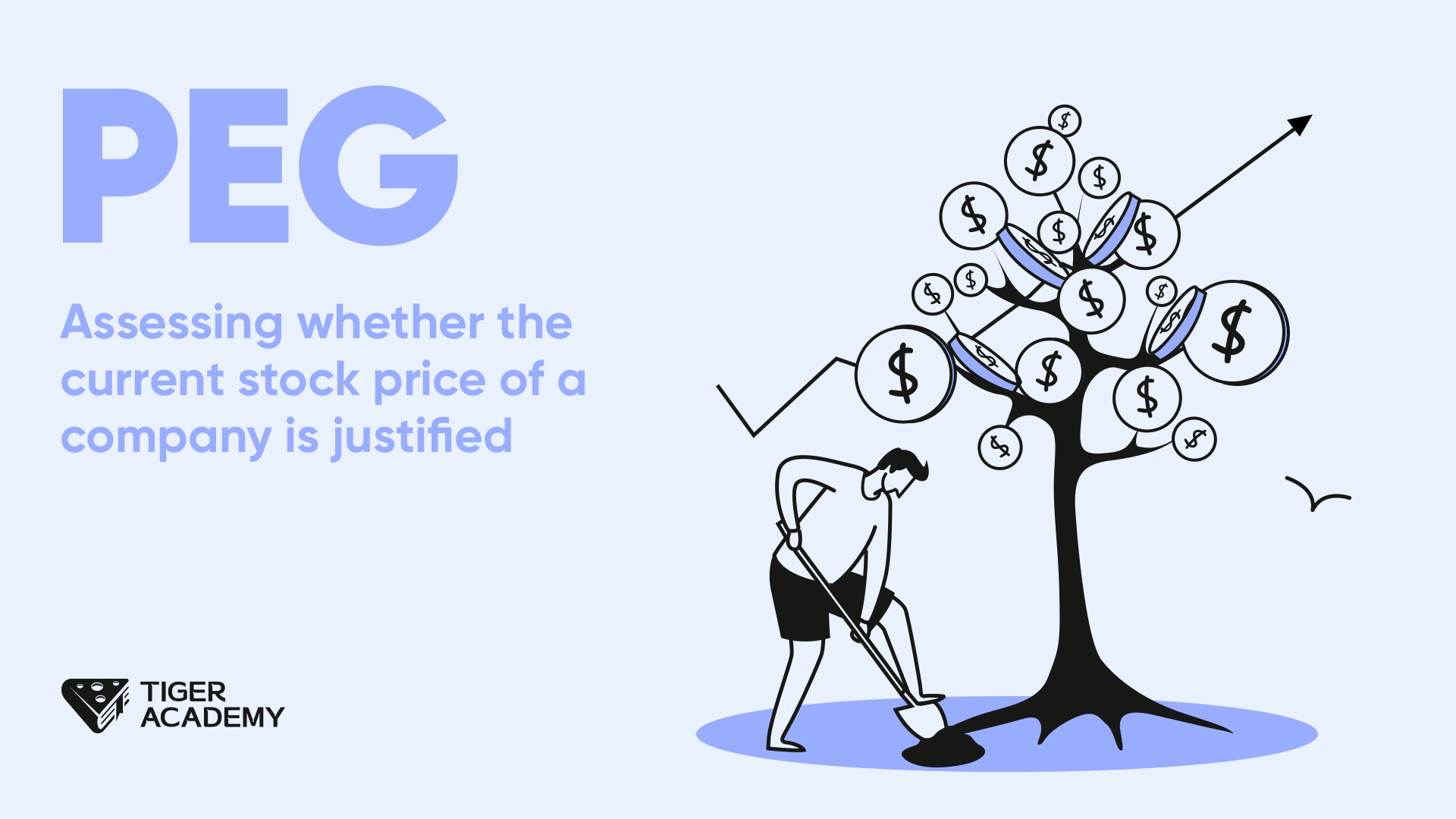## PEG

01 SepThe PEG ratio is a comprehensive indicator that combines a company's Price-to-Earnings ratio (PE) and earnings growth rate (EPS).

Specifically, the PEG ratio is the ratio of PE to EPS growth rate.

The PEG ratio is often used to help investors understand a company's growth prospects and whether its current stock price is reasonable.

If a company has a PEG ratio less than 1, it means that its stock price is reasonably valued relative to its earnings growth potential, indicating a favorable investment opportunity.

Conversely, if the PEG ratio is greater than 1, the company's stock price may be overvalued, and there might be some future risks.

Here's an example:

Suppose you are considering buying stocks of a company with a PE ratio of 25 and an expected annual growth rate of 20%. You can use the PEG ratio to assess whether the company is undervalued or overvalued.

The formula for calculating the PEG ratio is PE ratio divided by the annual growth rate. In this case, the PEG ratio would be 1.25 (25/20).

According to the PEG ratio guidelines, a company with a PEG ratio less than 1 is considered undervalued, while a PEG ratio greater than 1 indicates overvaluation. A PEG ratio equal to 1 is considered a reasonable valuation.

In this example, the company has a PEG ratio of 1.25, slightly higher than 1, which means the company is considered overvalued. In such a case, you may consider waiting for a price drop before buying or explore stocks of other companies.

Regarding the PEG ratio, here are some additional points to note:

1. The PEG ratio is only a reference indicator and should not be the sole basis for investment decisions. It needs to be considered along with other factors such as a company's financial condition and industry outlook.

2. Calculating the PEG ratio requires reliable future growth projections. Deviations in the projections can render the PEG ratio less meaningful as a reference.

3. PEG ratio standards may vary across different industries. You should compare the PEG ratio with companies in the same industry to determine if it holds investment value.

4. Pay attention to the company's earnings quality and stability. Do not solely rely on a low PEG ratio and overlook potential risks associated with the company.

In conclusion, the PEG ratio is a valuable reference indicator that can help you identify potential investment opportunities.

However, it should not be the sole basis for investment decisions and needs to be considered along with various factors!